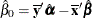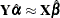### Implicit and Explicit Intercepts

Depending on several options, the model intercept is nonzero, zero, or implicit, or there is no intercept. Ordinarily, the model contains an explicit nonzero intercept, and the Intercept variable in the OUT= data set contains ones. When TSTANDARD=CENTER or TSTANDARD=Z is specified, the model contains an explicit, zero intercept and the Intercept variable contains zeros. When METHOD=CANALS, the model is fit with centered variables and the Intercept variable is set to missing.

If you specify CLASS with ZERO=NONE or BSPLINE for one or more independent variables, and TSTANDARD=NOMISS or TSTANDARD=ORIGINAL (the default), an implicit intercept model is fit. The intercept is implicit in a set of the independent variables since there exists a set of independent variables the sum of which is a column of ones. All statistics are mean corrected. The implicit intercept is not an option; it is implied by the model. Specifying SMOOTH or PBSPLINE also implies an implicit intercept model.

With METHOD=CANALS, the Intercept variable contains the canonical intercept for canonical coefficients observations:where.